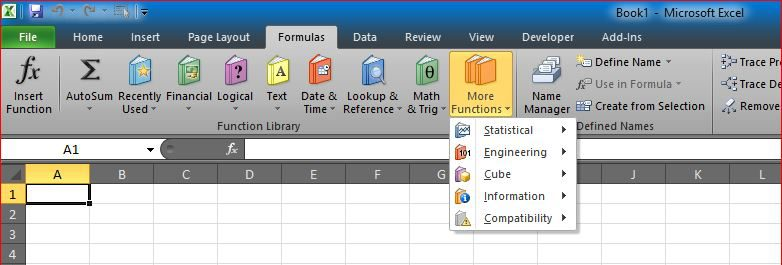# Calculate hours between two cells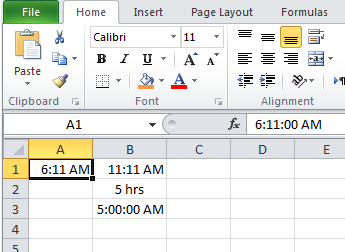We needed that several times in the past
Then I found it in an old file in my laptop, thought to share it for others to help

So, when A1 has the start time, B1 has the end time
Time different in one day will be from formula

`=IF(OR(A1="",B1=""),"",ABS((HOUR(A1)+MINUTE(A1)/60)-(HOUR(B1)+MINUTE(B1)/60))&" hrs")`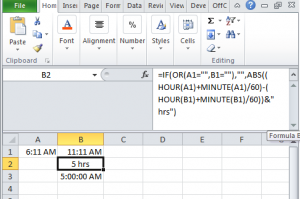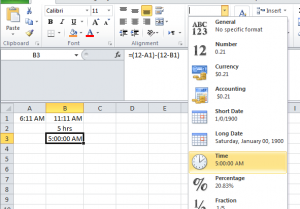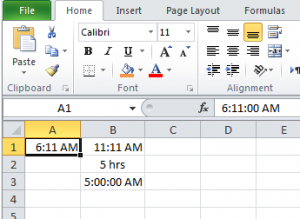I added to prevent having -ve number of hours in case start time and end times switched between A1 and B1